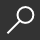How to Solve Word Problems in Calculus by Eugene Don and Benay Don

Overview -
Considered to be the hardest mathematical problems to solve, word problems continue to terrify students across all math disciplines. This new title in the World Problems series demystifies these difficult problems once and for all by showing even the most math-phobic readers simple, step-by-step tips and techniques. How to Solve World Problems in Calculus reviews important concepts in calculus and provides solved problems and step-by-step solutions. Once students have mastered the basic approaches to solving calculus word problems, they will confidently apply these new mathematical principles to even the most challenging advanced problems. Each chapter features an introduction to a problem type, definitions, related theorems, and formulas. Topics range from vital pre-calculus review to traditional calculus first-course content. Sample problems with solutions and a 50-problem chapter are ideal for self-testing. Fully explained examples with step-by-step solutions.

local_shippingFor DeliveryIn Stock.
FREE Shipping for Club Members helpNew & Used Marketplace 13 copies from \$3.45

More About How to Solve Word Problems in Calculus by Eugene Don; Benay Don

Overview

Considered to be the hardest mathematical problems to solve, word problems continue to terrify students across all math disciplines. This new title in the World Problems series demystifies these difficult problems once and for all by showing even the most math-phobic readers simple, step-by-step tips and techniques. How to Solve World Problems in Calculus reviews important concepts in calculus and provides solved problems and step-by-step solutions. Once students have mastered the basic approaches to solving calculus word problems, they will confidently apply these new mathematical principles to even the most challenging advanced problems. Each chapter features an introduction to a problem type, definitions, related theorems, and formulas. Topics range from vital pre-calculus review to traditional calculus first-course content. Sample problems with solutions and a 50-problem chapter are ideal for self-testing. Fully explained examples with step-by-step solutions.

Details

• ISBN-13: 9780071358972
• ISBN-10: 0071358978
• Publisher: McGraw-Hill Companies
• Publish Date: July 2001
• Page Count: 240
• Dimensions: 8.55 x 5.55 x 0.52 inches
• Shipping Weight: 0.68 pounds

Series: How to Solve Word ProblemsRelated Categories